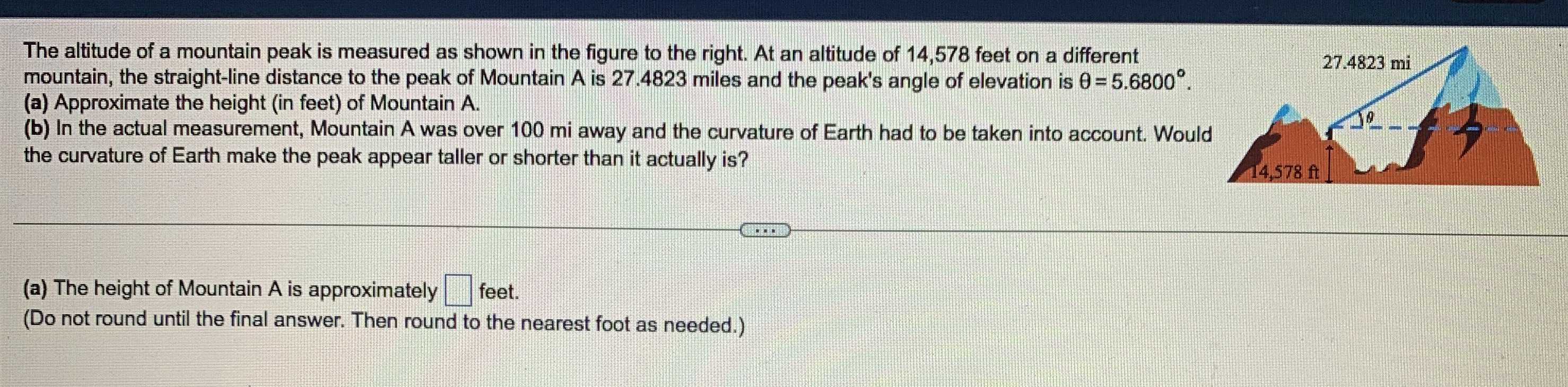### Still have math questions?

Trigonometry
QuestionThe altitude of a mountain peak is measured as shown in the figure to the right. At an altitude of $$14,578$$ feet on a different mountain, the straight-line distance to the peak of Mountain $$A$$ is $$27.4823$$ miles and the peak's angle of elevation is $$\theta = 5.6800 ^ { \circ }$$ . Approximate the height (in feet) of Mountain A.